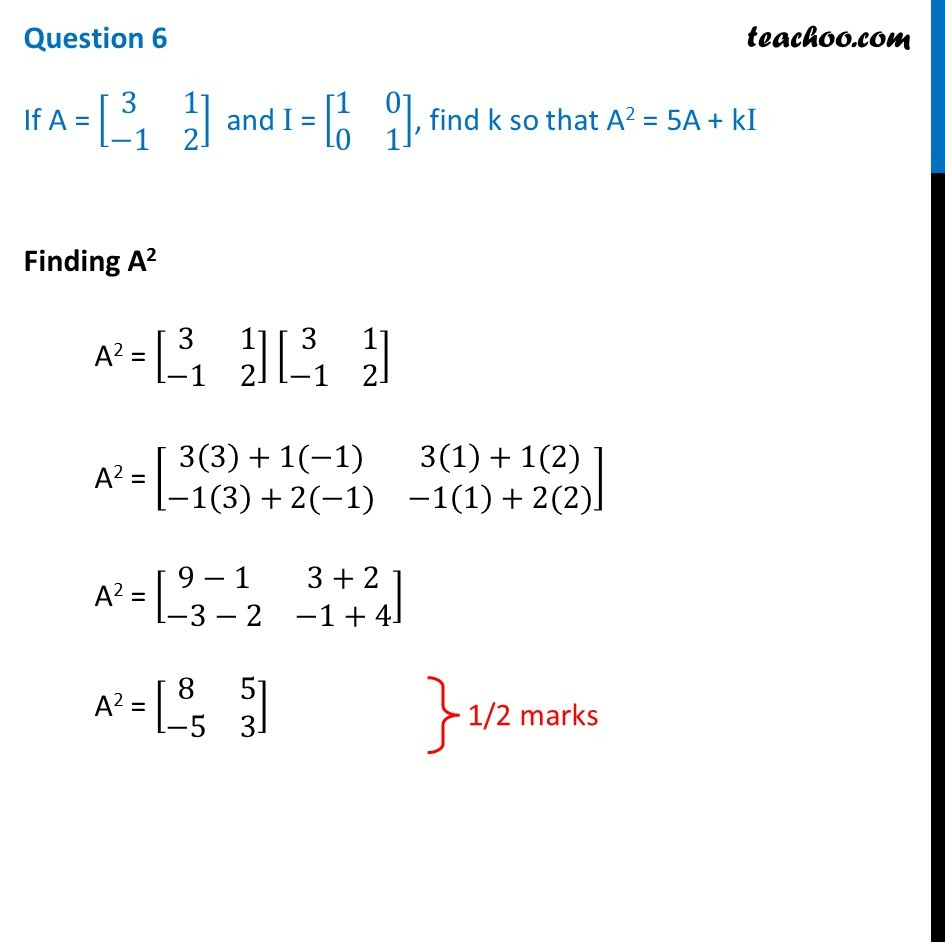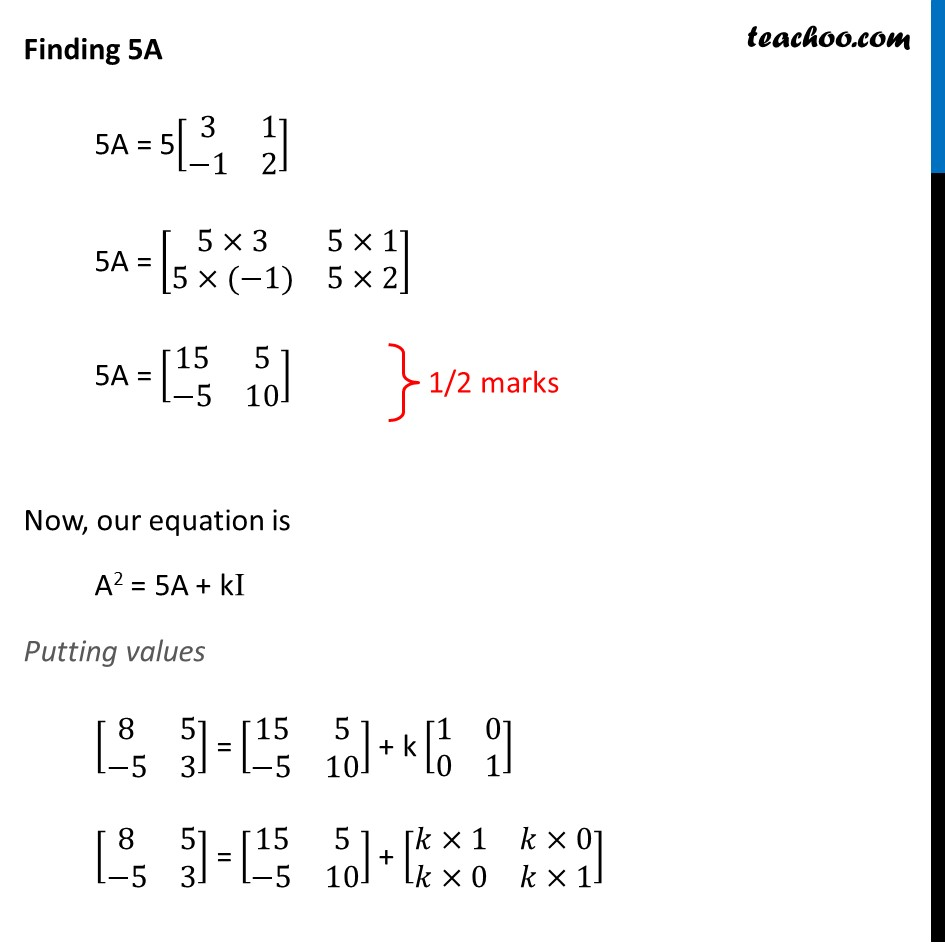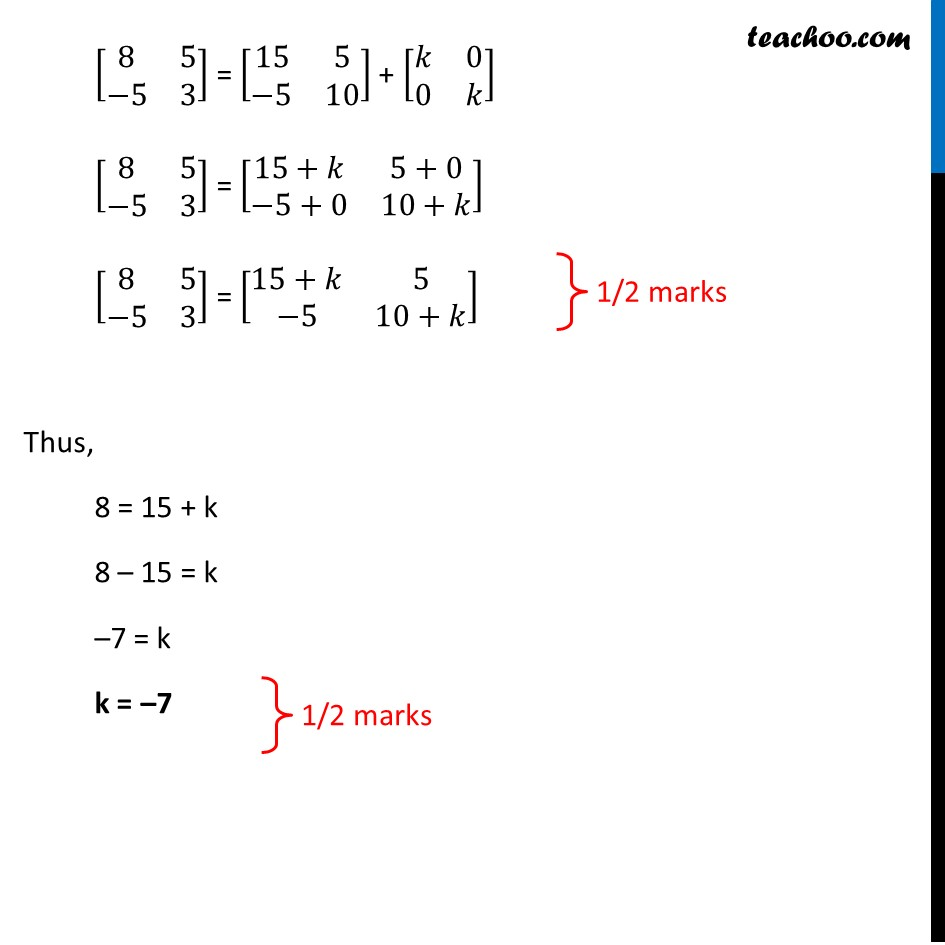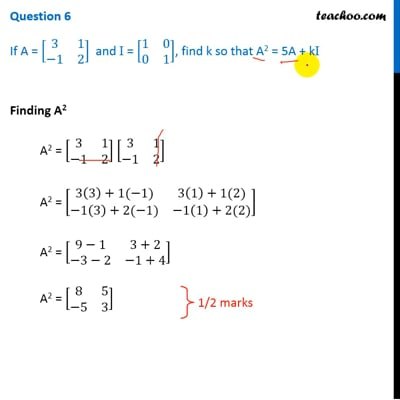CBSE Class 12 Sample Paper for 2019 Boards

Class 12
Solutions of Sample Papers and Past Year Papers - for Class 12 Boards

Question 6

If A = [3 1 -1 2] and I = [1 0 0 1], find k so that A 2 = 5A + kIThis video is only available for Teachoo black users

Learn in your speed, with individual attention - Teachoo Maths 1-on-1 Class

### Transcript

Question 6 If A = [■8(3&1@−1&2)] and I = [■8(1&0@0&1)], find k so that A2 = 5A + kI Finding A2 A2 = [■8(3&1@−1&2)] [■8(3&1@−1&2)] A2 = [■8(3(3)+1(−1)&3(1)+1(2)@−1(3)+2(−1)&−1(1)+2(2))] A2 = [■8(9−1&3+2@−3−2&−1+4)] A2 = [■8(8&5@−5&3)] Finding 5A 5A = 5[■8(3&1@−1&2)] 5A = [■8(5×3&5×1@5×(−1)&5×2)] 5A = [■8(15&5@−5&10)] Now, our equation is A2 = 5A + kI Putting values [■8(8&5@−5&3)] = [■8(15&5@−5&10)] + k [■8(1&0@0&1)] [■8(8&5@−5&3)] = [■8(15&5@−5&10)] + [■8(𝑘×1&𝑘×0@𝑘×0&𝑘×1)] [■8(8&5@−5&3)] = [■8(15&5@−5&10)] + [■8(𝑘&0@0&𝑘)] [■8(8&5@−5&3)] = [■8(15+𝑘&5+0@−5+0&10+𝑘)] [■8(8&5@−5&3)] = [■8(15+𝑘&5@−5&10+𝑘)] Thus, 8 = 15 + k 8 – 15 = k –7 = k k = –7